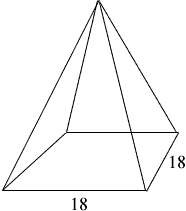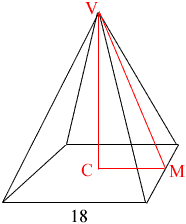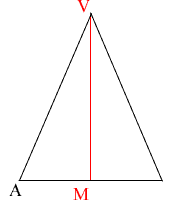Quandaries and Queries My name is Jacob and I am a 11th grade student trying to build a pyramid for a friend but I need a chart of some sort to show me the measurements (I learn better w\pictures) it is a square base with 18 in sides and I want the vertex to be 18in above the base I need to know as many measurements as you can give me. Thank You! Hi Jacob, I learn better with pictures myself but I learn best when I draw my own pictures. Draw a picture of your pyramid.I expect that your friend wants a regular pyramid, that is one where the four triangular sides are congruent. The vertex of the pyramid is then directly above the center of the square base so draw a perpendicular line from the vertex V to the center C of the base, join the center C of the base to the middle M of one of the sides of the square and then complete a triangle by joining this point M to the vertex V.The triangle VCM is a right triangle with |VC| = 18 and |CM| = 9 so you can use Pythagoras' theorem to find |VM|. Now look at the face containing the line segment VM.VAM is a right triangle, |VM| you just found and |AM| = 9, hence you can use Pythagoras' Theorem again, this time to find |VA|. Penny Go to Math Central